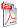Issue archive

https://doi.org/10.15255/KUI.2021.001
Published: Kem. Ind. 70 (11-12) (2021) 675–691
Paper reference number: KUI-01/2021
Paper type: Original scientific paper
Download paper:PDFOptimisation and Prediction of the Coagulant Dose for the Elimination of Organic Micropollutants Based on Turbidity

H. Tahraoui, A. E. Belhadj, N. Moula, S. Bouranene and A. Amrane

##### Abstract

In this study, four different mathematical models were considered to predict the coagulant dose in view of turbidity removal: response surface methodology (RSM), artificial neural networks (ANN), support vector machine (SVM), and adaptive neuro-fuzzy inference system (ANFIS). The results showed that all models accurately fitted the experimental data, even if the ANN model was slightly above the other models. The SVM model led to almost similar results as the ANN model; the only difference was in the validation phase, since the correlation coefficient was very high and the statistical indicators were very low for the ANN model compared to the SVM model. However, from an economic point of view, the SVM model was more appropriate than the ANN model, since its number of parameters was 22, i.e., almost half the number of parameters of the ANN model (43 parameters), while the results were almost similar in all the data phase. To reduce the economic costs further, the RSM model can also be used, which remained very useful due to its high coefficients related to the number of parameters – only 13. In addition, the statistical indicators of the RSM model remained acceptable.

##### Keywords

coagulation, physicochemical analysis, response surface methodology, artificial neural networks, support vector machine, adaptive neuro-fuzzy inference system